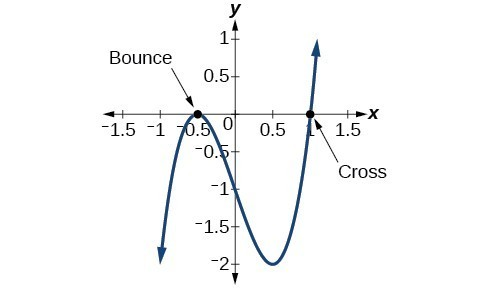## Find zeros of a polynomial function

The Rational Zero Theorem helps us to narrow down the list of possible rational zeros for a polynomial function. Once we have done this, we can use synthetic division repeatedly to determine all of the zeros of a polynomial function.

### How To: Given a polynomial function $f$, use synthetic division to find its zeros.

1. Use the Rational Zero Theorem to list all possible rational zeros of the function.
2. Use synthetic division to evaluate a given possible zero by synthetically dividing the candidate into the polynomial. If the remainder is 0, the candidate is a zero. If the remainder is not zero, discard the candidate.
3. Repeat step two using the quotient found with synthetic division. If possible, continue until the quotient is a quadratic.
4. Find the zeros of the quadratic function. Two possible methods for solving quadratics are factoring and using the quadratic formula.

### Example 5: Finding the Zeros of a Polynomial Function with Repeated Real Zeros

Find the zeros of $f\left(x\right)=4{x}^{3}-3x - 1$.

### Solution

The Rational Zero Theorem tells us that if $\frac{p}{q}$ is a zero of $f\left(x\right)$, then is a factor of –1 and q is a factor of 4.

$\begin{cases}\frac{p}{q}=\frac{\text{factor of constant term}}{\text{factor of leading coefficient}}\hfill \\ \text{ }=\frac{\text{factor of -1}}{\text{factor of 4}}\hfill \end{cases}$

The factors of –1 are $\pm 1$ and the factors of 4 are $\pm 1,\pm 2$, and $\pm 4$. The possible values for $\frac{p}{q}$ are $\pm 1,\pm \frac{1}{2}$, and $\pm \frac{1}{4}$.
These are the possible rational zeros for the function. We will use synthetic division to evaluate each possible zero until we find one that gives a remainder of 0. Let’s begin with 1.

Dividing by $\left(x - 1\right)$ gives a remainder of 0, so 1 is a zero of the function. The polynomial can be written as

$\left(x - 1\right)\left(4{x}^{2}+4x+1\right)$.

The quadratic is a perfect square. $f\left(x\right)$ can be written as

$\left(x - 1\right){\left(2x+1\right)}^{2}$.

We already know that 1 is a zero. The other zero will have a multiplicity of 2 because the factor is squared. To find the other zero, we can set the factor equal to 0.

$\begin{cases}2x+1=0\hfill \\ \text{ }x=-\frac{1}{2}\hfill \end{cases}$

The zeros of the function are 1 and $-\frac{1}{2}$ with multiplicity 2.

### Analysis of the Solution

Look at the graph of the function f in Figure 1. Notice, at $x=-0.5$, the graph bounces off the x-axis, indicating the even multiplicity (2,4,6…) for the zero –0.5. At $x=1$, the graph crosses the x-axis, indicating the odd multiplicity (1,3,5…) for the zero $x=1$.Figure 1